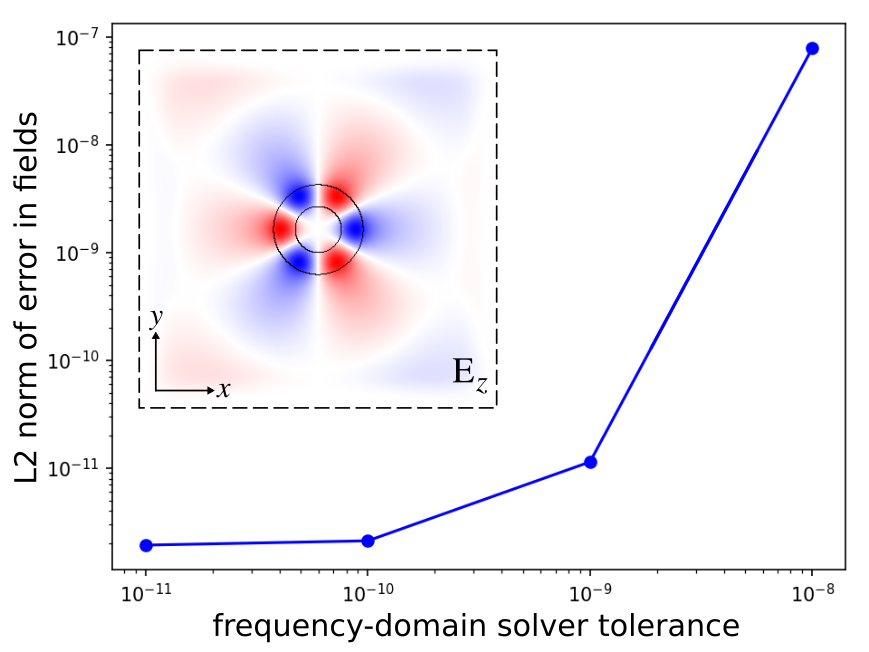# Frequency Domain Solver

This tutorial demonstrates Meep's frequency-domain solver which is used to compute the fields produced in a geometry in response to a continuous-wave (CW) source. For details on how this feature works, see Section 5.3 ("Frequency-domain solver") of Computer Physics Communications, Vol. 181, pp. 687-702, 2010. This example involves using the frequency-domain solver to compute the fields of a ring resonator which has been described in Tutorial/Basics/Modes of a Ring Resonator. We will verify that the error in the computed fields decreases monotonically with decreasing tolerance of the iterative solver.

Usage of the frequency-domain solver involves only two changes to the original simulation: (1) replace the Gaussian-pulse source with a continuous source, and (2) turn on complex fields since, by default, real fields are used. Everything else remains unchanged.

Since the frequency-domain solver uses an iterative method, there are a couple of things we can do to improve its convergence: (1) use a non-zero smoothing width for the CW source (default is 0) to reduce the high-frequency oscillations produced by its abrupt turn on (which have slow group velocities and are absorbed poorly by PML), and (2) increase the $L$ parameter of the BiCGSTAB-L iterative solver from the default of 2 to 10.

We will compute the fundamental mode at five different tolerance values chosen on a logarithmic scale: 1e-8, 1e-9, 1e-10, 1e-11, 1e-12. We will then plot the L2 norm of the error in the fields (relative to the results at a tolerance of 1e-12) as a function of the tolerance.

The simulation script is in examples/solve-cw.ctl.

(define-param n 3.4)
(define-param w 1)
(define-param r 1)
(define-param dpml 2)

(define sxy (* 2 (+ r w pad dpml)))
(set! geometry-lattice (make lattice (size sxy sxy no-size)))

(set! geometry (list
(make cylinder (center 0 0) (height infinity)
(radius (+ r w)) (material (make dielectric (index n))))
(make cylinder (center 0 0) (height infinity)

(set! pml-layers (list (make pml (thickness dpml))))
(set-param! resolution 10)

(define-param fcen 0.118)
(set! sources (list
(make source
(src (make continuous-src (frequency fcen)))
(component Ez)
(center (+ r 0.1) 0))
(make source
(src (make continuous-src (frequency fcen)))
(component Ez)
(center (- (+ r 0.1)) 0)
(amplitude -1))))

(set! symmetries (list (make mirror-sym (direction X) (phase -1))
(make mirror-sym (direction Y) (phase +1))))

(set! force-complex-fields? true)

(define-param solve-cw-tol 1e-8)
(define-param solve-cw-maxiters 10000)
(define-param solve-cw-L 10)

(define (ez-real r ez) (real-part ez))

(init-fields)
(meep-fields-solve-cw fields solve-cw-tol solve-cw-maxiters solve-cw-L)
(in-volume (volume (center 0 0) (size (- sxy (* 2 dpml)) (- sxy (* 2 dpml))))
(output-epsilon)
(output-real-field-function "ez-real" (list Ez) ez-real))

(exit)


The results are shown in the figure below. The field profile is generated using h5utils: h5topng -o ring_field_profile.png -vZc bluered -C solve-cw-eps-000000.00.h5 solve-cw-ez-real-000000.00.h5. The error in the fields decreases monotonically with decreasing tolerance of the frequency-domain solver. The error is converging to an asymptotic limit of 1e-12 which is set by the lowest tolerance. The inset shows the real part of the scalar Ez field, computed using a tolerance of 1e-12, superimposed on the ring-resonator geometry. Note the three-fold mirror symmetry of the field pattern (fundamental mode) and the faint presence of the point source.